Working out chord and scale transpositions can be a bit frustrating. You could learn all the chords and scales in every fret position for each string, you could use a book of scales and chords, or you could try this Guitar Chord and Scale Translator.

It names the notes by their position relative to a root note, placed on a fretboard, and uses the named notes along with either a chord or scale formulae chart.

A brief description of the diagrams is provided below, followed by some examples.

### The Fretboard Diagram

The fretboard diagram is a reference for working out where to put your fingers on the fretboard when using either the chord or scale formulae.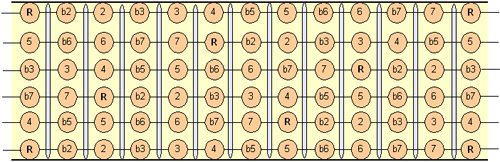Fretboard

R corresponds to the Root note of a scale or chord.

#### Chord Formulae Diagram

The chart below contains the basic chord formulae. Each row is a separate chord, showing the individual notes that make up each chord.Chord Formulae

Use the note names relevant to the type of chord you want to play, work out where those notes are on the fretboard. Make sure a note from the formula for a particular chord is assigned to each played string.

#### Scale Formulae Diagram

The chart below contains the basic scale formulae. Each row is a separate scale, showing the individual notes that make up each scale.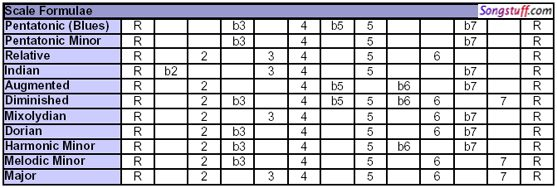Music Scale Formulae

Use the note names relevant to the type of scale you want to play, work out where those notes are on the fretboard.

#### Example: Major Chord

Use the Major Chord formula from the Chord Formulae Diagram: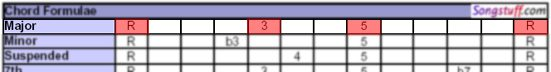Major Chord Formula

Work out where those notes lie on the fretboard using the Fretboard Diagram. If the chord in question is an E Major, then the Root Note is an E, which is also an open string on a guitar. The bottom of the fretboard diagram corresponds to a low E on the Guitar (The 6th string).

The notes for an E major, where the root note is on the sixth string could look like this.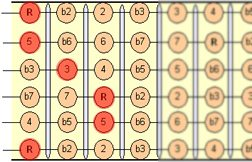Major Chord

Try the same E Major Chord in different positions on the neck.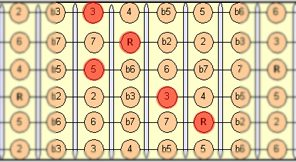Relocated Major Chord

#### Example: Major Scale

Use the Major Scale formula from the Scale Formulae Diagram: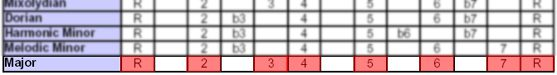Major Scale Formula

Work out where those notes lie on the fretboard using the Fretboard Diagram. The bottom of the fretboard diagram corresponds to a low E on the Guitar (The 6th string).Major Scale

Try the different fingering options for the same scale.Relocation of Major Scale

Several times...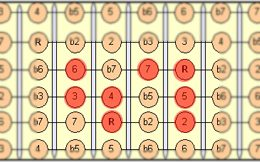Relocation of a Major Scale (2)

Drop by the Songstuff Forums to discuss guitars and to discuss this article.